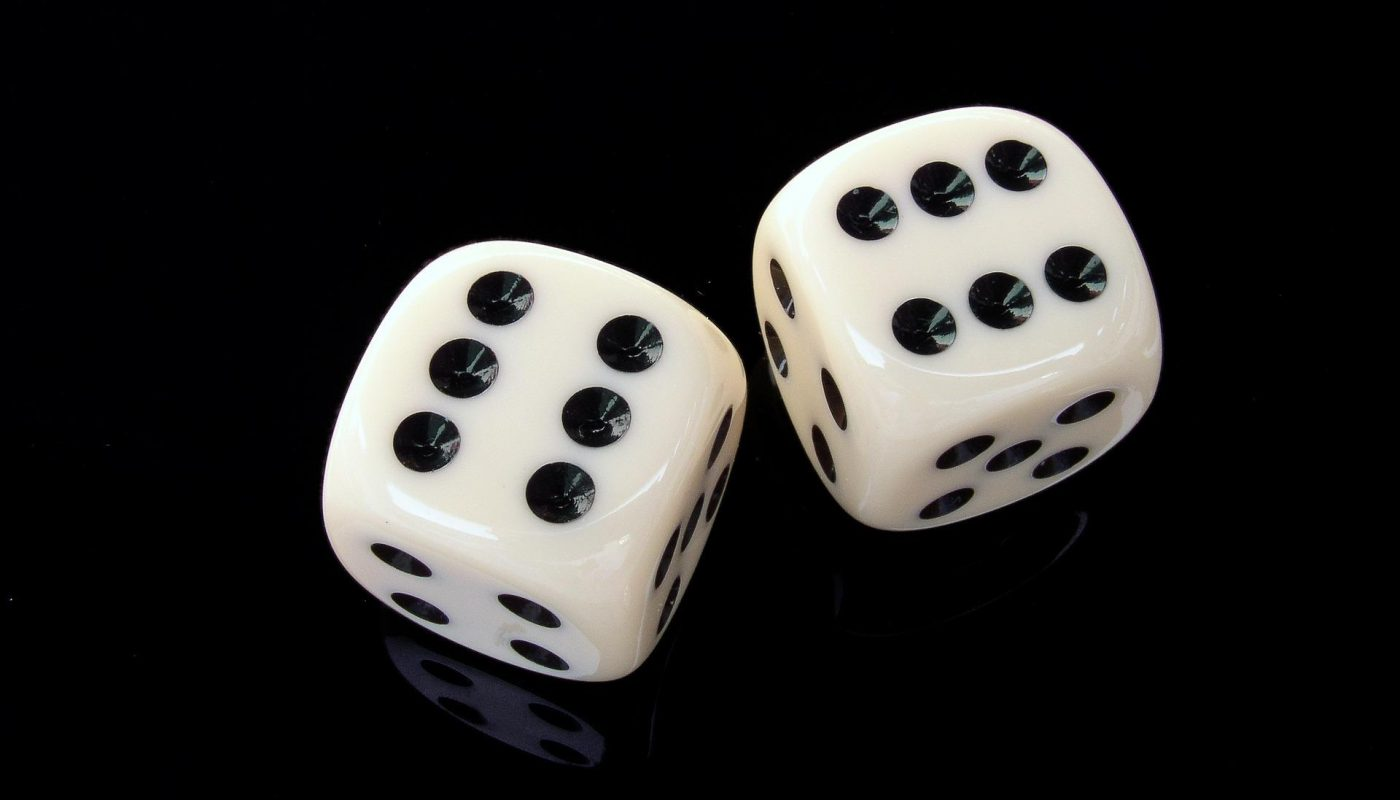# Betting Odds Explained: A Beginner’s Guide

The desire to make money by predicting games is growing, especially among young people. In India, sports has become one of the fastest means to make money and that is why it appears many are considering a professional gaming career. But unfortunately, many people who want to predict games ignore the fundamentals. No matter how small or big a building will be, attention has to be paid to the foundation. The building will not stand when the foundation is faulty, the building will not stand, which applies to sports predictions.

If you’re new to sports prediction in India, there’s a lot you need to familiarize yourself with. Some of the most important are gaming odds; understanding how these odds work is the first step. Understanding gaming odds is extremely important in sports predictions because they tell you how likely it is for an event to happen. They also give you pointers on what your potential winnings will be.

Betting odds will appear complicated because you’re new to making predictions, but we will simplify them in this article. That includes explaining what the formats Decimal and Functional mean in gaming odds.

## The Fractional Format: Using Odds to Calculate Probability

You’re probably wishing you took math seriously in school; don’t worry, many of us didn’t. The simplest gift betting gives you is the ability to predict an event’s outcome; if you’re right, you’ll win real money. There’s a specific number of outcomes for any sporting event–like when you roll a dice, you know there are six possible outcomes. So, if you bet that the person rolls a “4,” there is a 16.67 chance it will happen.

Fractional odds presented as two numbers with a slash between them – for example, A/B; you calculate the possibility of an event happening. So let’s do some sports-betting-related math, shall we?

Gaming odds are calculated as a probability (%) = B/(A+B)

So, if an event has 9/1 odds, you calculate that as 1 / (9+1) = 1/10 = 0.10 – so, there’s a ten percent chance that this event will happen.

Let’s try some other examples:

1/4 = 4 / (1+4) = 4/5 = 0.80 – there’s an eight percent chance that his particular event will happen.

2/2 = 2 / (2+2) = 2/4 = 0.50 – there’s a fifty percent chance that this particular event will happen.

So, we believe you know how to tell the likelihood of an event happening using fractional betting odds. You may not find this information clear until you start predicting games. However, some gaming sites try to simplify the fractional odds for users to help them understand it and prevent panic predictions.

## Using Fractional Betting Odds to Calculate Winnings

When predicting a game, many things have to be done after deciding the game you want to play. One such thing is calculating the odds and deciding whether that game is worth putting your money in. The higher the odds, the better your chances of getting more money if the game goes your way. Conversely, if the odds are low, you will have to put in more money to get something out of today match odds.

Betting odds help you calculate how much you’ll win if you bet on an outcome and it happens. Using the examples we used before, A/B, let’s calculate the value for each bet and the return of your stake.

In 9/1, for every 1 rupee you bet, you’ll gain 9 rupees,

In 1/4, for every 4 rupees you bet, you get 1 rupee, and

In 2/2, for every 2 rupees you bet, you get 2 rupees.

## The Decimal Format of Betting Odds

Decimal betting odds formats are more popular on betting exchanges than the fractional format. You will find more gaming platforms that favor decimal formats because of how easy it is for everyone to understand. However, all top betting sites in India let you view betting odds in decimal formats; they are an easier alternative to fractional formats.

Calculating these odds is (odds * stake) – stake. Let’s practicalize the decimal format with some examples, too;

• You can calculate 8.0 odds as (8.0 * 10 rupees stake) – 10 rupees stake = 80 – 10 = 70 rupees winnings.
• You can calculate 1.5 odds as (1.5 * 10 rupees stake) – 10 rupees stake = 15 – 10 = 5 rupees winnings
• You can calculate 3.0 odds as (3.0 * 10 rupees stake) – 10 rupees stake = 30 – 10 = 20 rupees winnings.

## Conclusion

We’ve broken down betting odds as simply as possible; we believe you understand them now. You also understand that betting odds present the likelihood or probability that an event will happen. That, in turn, enables you to calculate your winnings if the event you predicted happens. You should now have a good knowledge of betting odds, their importance, and how to calculate your winnings in the two formats.

Inside Contents#### Sonal Shukla

View all posts by Sonal Shukla →